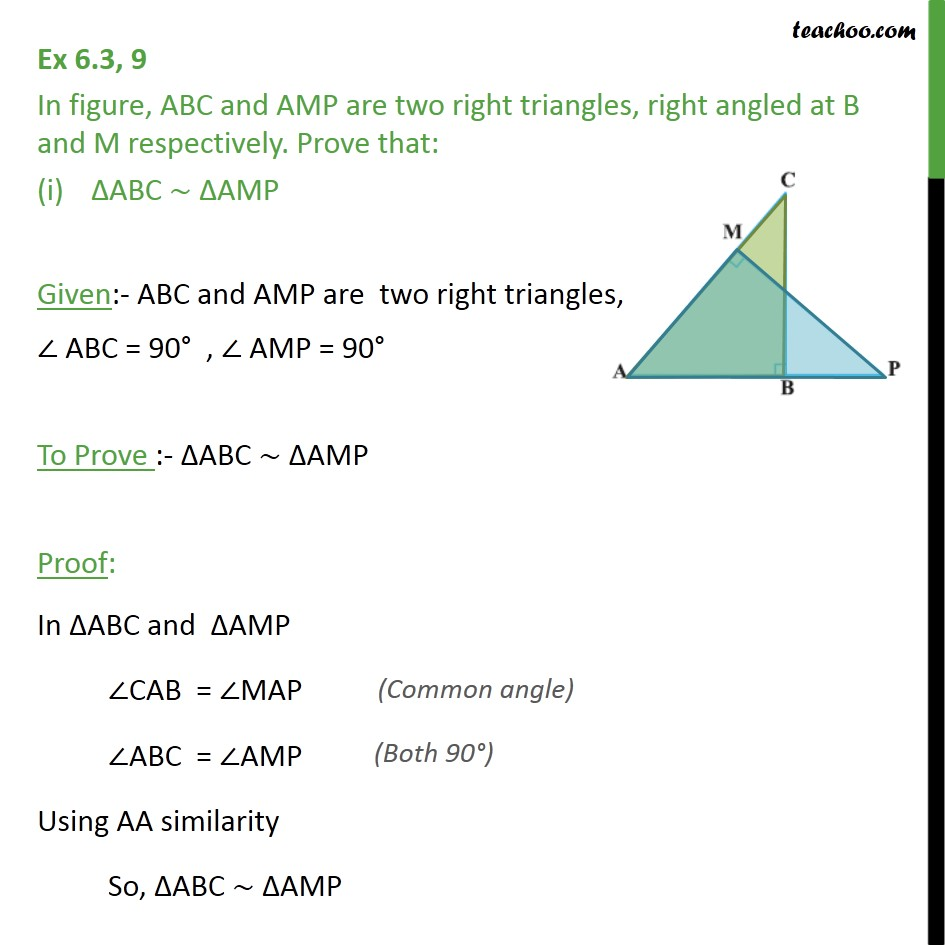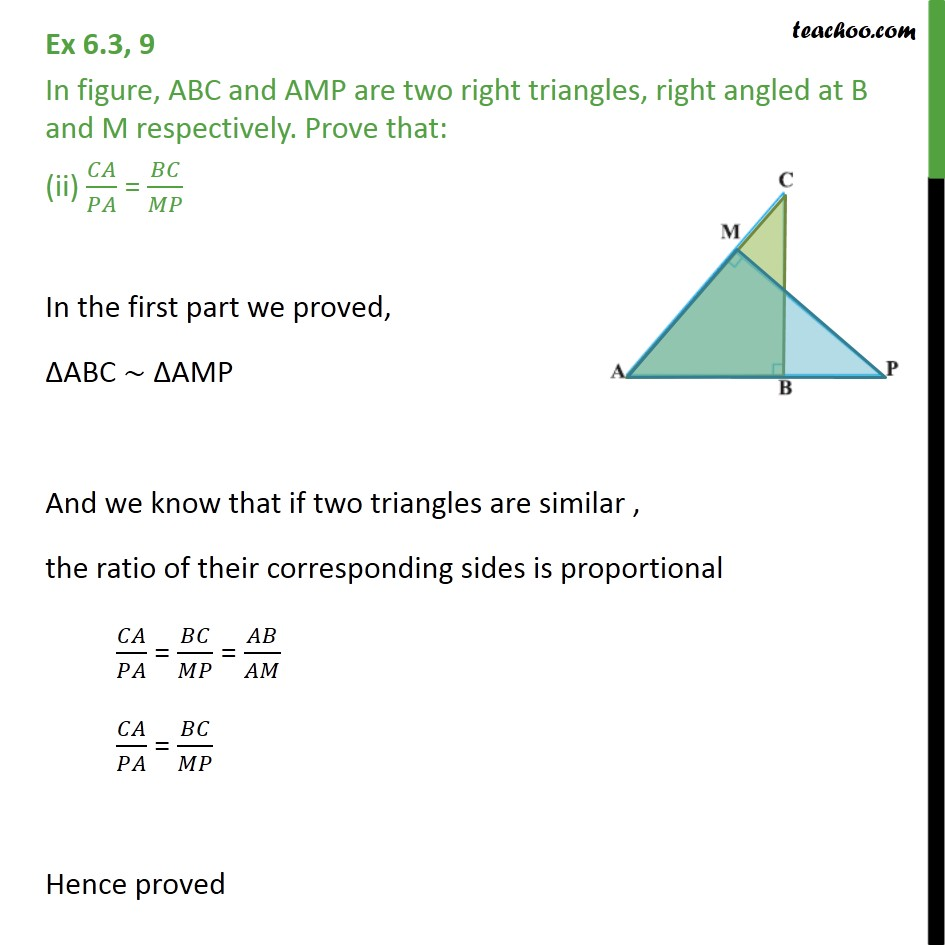.Subscribe to our Youtube Channel - https://you.tube/teachoo

1. Chapter 6 Class 10 Triangles
2. Concept wise
3. AA Similarity

Transcript

Ex 6.3, 9 In figure, ABC and AMP are two right triangles, right angled at B and M respectively. Prove that: ΔABC ∼ ΔAMP Given:- ABC and AMP are two right triangles, ∠ ABC = 90° , ∠ AMP = 90° To Prove :- ΔABC ∼ ΔAMP Proof: In ΔABC and ΔAMP ∠CAB = ∠MAP ∠ABC = ∠AMP Using AA similarity So, ΔABC ∼ ΔAMP Ex 6.3, 9 In figure, ABC and AMP are two right triangles, right angled at B and M respectively. Prove that: (ii) 𝐶𝐴/𝑃𝐴 = 𝐵𝐶/𝑀𝑃 In the first part we proved, ΔABC ∼ ΔAMP And we know that if two triangles are similar , the ratio of their corresponding sides is proportional 𝐶𝐴/𝑃𝐴 = 𝐵𝐶/𝑀𝑃 = 𝐴𝐵/𝐴𝑀 𝐶𝐴/𝑃𝐴 = 𝐵𝐶/𝑀𝑃 Hence proved

AA Similarity